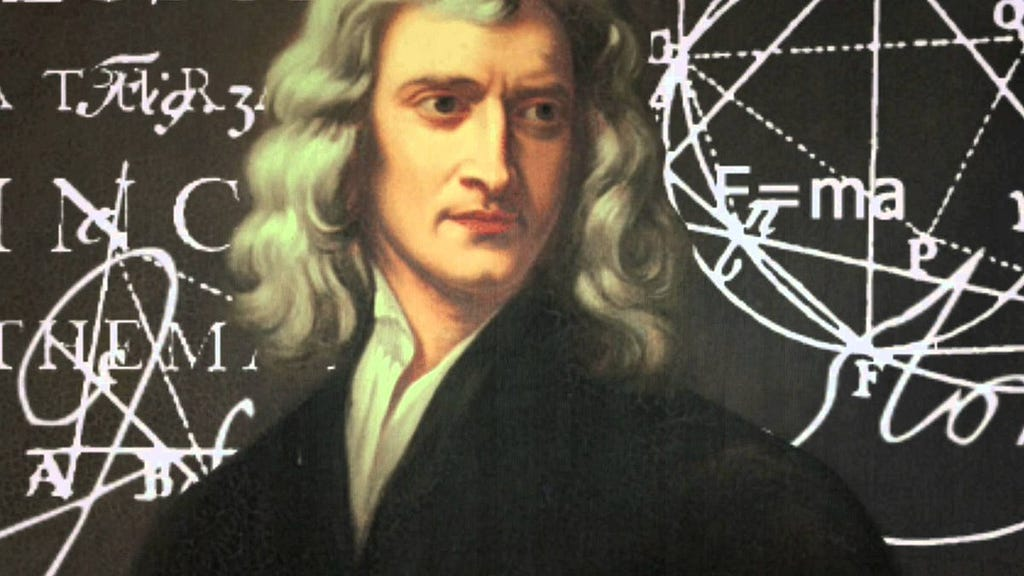Join thousands of AI enthusiasts and experts at the Learn AI Community.

## PublicationLatest

# How To Discover The Laws Of Physics With Deep Learning and Symbolic Regression

Last Updated on September 14, 2021 by Editorial Team

### Scientist vs Machine Learning

I am turned into a sort of machine for observing facts and grinding out conclusions.” — Charles Darwin

Science cannot work without data. Similarly, machine learning cannot work without data. The scientific method and machine learning are very close to each other.

To understand the universe, scientists identify a problem and collect data about it. From the collected data, scientists create models and evolve this process by constantly creating better models. Machine learning also tries to create the best model with the data it collects from the environment. It’s a model you have in your hand.

The human mind has limited processing power and memory. Artificial intelligence presents a new regime of scientific inquiry, where we can automate the research process itself. AI will lead to a paradigm shift in scientific research.

But there are some problems that we need to solve first. Why are Maxwell’s equations considered a science fact, but a deep learning model just a black box? Here, interpretability and generalizability begin to play an important role.

Later in the article, we will see how high-dimensional data is compressed into analytical equations.

#### Golden Rules

Everything should be made as simple as possible but not simpler -Einstein

Interpretability and generalizability are the golden rules for scientific equations. The best example is F=m*a it is very interpretable and generalizable. You can use it to predict the motion of the apple and moon.

Much of the rules in the natural sciences are accurately described by simple symbolic equations. Such as Work, energy, motion, radiation,
fluid dynamics, thermodynamics. Unreasonable effectiveness in mathematics encourages us to solve complex problems with simple equations.

If you train a deep learning model to predict the falling of apples probably, it won’t be helpful to use this model in space missions. Deep learning is not the solution to every problem.

Deep learning proves extraordinarily efficient at learning in high-dimensional spaces but suffers from poor generalization and interpretability. On the other hand, Symbolic Regression is very good at generalization, but it isn’t very good at high-dimensional data.

So, does there exist a way to combine the strengths of both?

### Learning Mechanics

The Neural Network's role in our approach is to anticipate targets and split them down into tiny internal functions that operate in low-dimensional spaces. Symbolic regression then utilizes an analytic equation to approximate the function of the deep model.

Studies have shown that GNNs are generally successful in learning physics problems. The message function of the GNN is analogous to a force, whereas the node update function is analogous to Newton’s law of motion.

We reduce the dimensionality of each function in order for the GNN to become sparse. Symbolic regression will be able to extract an expression better as a result of this.

#### Latent Space To Formula

Symbolic regression is a kind of regression analysis that looks for the best model in a space of mathematical expressions.

Mathematical building elements such as mathematical operators, analytic functions, constants, and state variables are randomly combined to create initial expressions. After that, an evolutionary method is used to improve these variables.

For example, a mutation can replace the + operator with ÷ or the variable x2 with x3.

The fitness function that drives model evolution considers error metrics and specific complexity measurements, ensuring that the final models explain the data’s underlying structure in a manner that is comprehensible to humans.

Symbolic Regression avoids imposing previous assumptions and infers the model from the data, while traditional regression methods attempt to maximize the parameters for a pre-specified model structure.

Genetic algorithms cannot successfully optimize the high-dimensional search space. We attempt to transform a deep neural network into a simplified mathematical equation to solve this issue.

While discovering the laws of physics, symbolic Regression does not learn from raw data, but it learns from what neural networks learned.

### Discovering Unknown

If we can rediscover dynamics that we know using ML, we can discover new dynamical systems that we do not know.

Cosmology is the study of the Universe’s development from the Big Bang to the complex structures we observe today, such as galaxies and stars. This evolution is driven by the interactions of different matter and energy, despite dark matter accounting for 85% of all matter in the Universe .

Dark matter particles cluster together to create dark matter halos, which serve as gravitational basins that draw matter together to form stars and bigger structures like galaxies.

The ability to deduce characteristics of dark matter halos from their surroundings is a crucial issue in cosmology. A halo’s overdensity was predicted by the same GNN model used before.

The algorithm developed has found an analytic equation that outperforms the one produced by scientists.

#### Useful Libraries

PySR(Parallelized symbolic regression)built on Julia and interfaced by Python. Uses regularized evolution, simulated annealing, and gradient-free optimization.

SINDY is a sparse regression package with several implementations for the Sparse Identification of Nonlinear Dynamical systems.

### Takeaways

In scientific research, new horizons will emerge due to the joint work of machine learning and human intelligence.

No technique is perfect. It has strengths and weaknesses. Combining methods correctly will be an essential part of advancing scientific research.

### References:

Thanks to Steve Brunton’s Youtube ChannelHow To Discover The Laws Of Physics With Deep Learning and Symbolic Regression was originally published in Towards AI on Medium, where people are continuing the conversation by highlighting and responding to this story.

Published via Towards AI